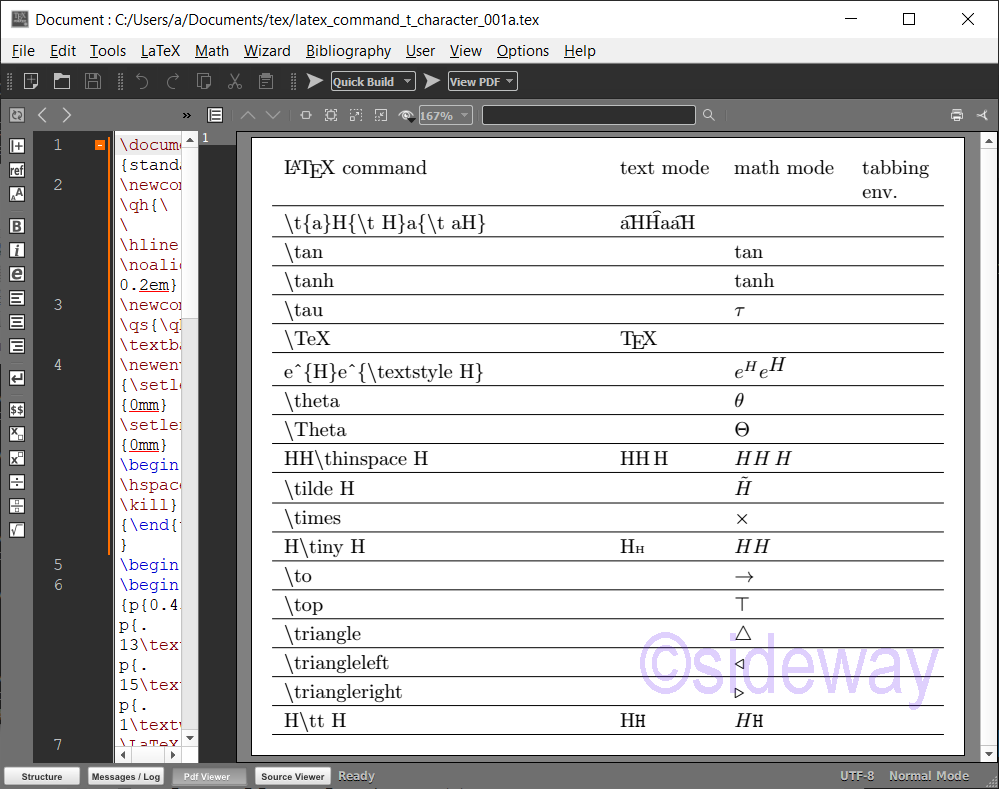output.to from Sideway
Draft for Information Only

# Content

LaTeX \t⋯ Commands for Printing Characters \t⋯ Commands \t⋯ Command Examples Source and Reference

# LaTeX \t⋯ Commands for Printing Characters

LaTeX uses backslash+t⋯ combination to print characters.

## \t⋯ Commands

The typical \t⋯ Commands for Printing Characters are LaTeX CommandsRemarksMode \tprints a tie-after accent, ⁀. \tanprints math, tan.math mode \tanhprints math, tanh.math mode \tauprints a Greek small letter tau, τ.math mode \TeXprints a TEX logo. \textstyleprints math environment typesetting characters.math mode \thetaprints a Greek small letter theta, θ.math mode \Thetaprints a Greek capital letter theta, Θ.math mode \tildeprints a above tilde character. e.g. ~, Ã, ã.math mode \timesprints a multiplication sign, ×.math mode \tinyprints very small typeface characters. \toprints a rightwards arrow, →. \topprints a down tack, ⊤. \triangleprints a white up-pointing triangle, △. \triangleleftprints a white left-pointing small triangle, ◃. \trianglerightprints a white right-pointing small triangle, ▹. \ttprints typewriter type characters.

## \t⋯ Command Examples

Examples of LaTeX commands
###### Code
\documentclass[border=10pt,varwidth]{standalone}
\newcommand\qh{\\\hline\noalign{\vskip 0.2em}}
\newcommand\qs{\qh\textbackslash}
\newenvironment{tabtest} {\setlength{\topsep}{0mm}\setlength{\partopsep}{0mm}\begin{tabbing}\hspace*{9mm}\kill}{\end{tabbing} }
\begin{document}
\begin{tabular}{p{0.45\textwidth}p{.13\textwidth}p{.15\textwidth}p{.1\textwidth}}
\LaTeX\ command & text mode & math mode & tabbing env.
\qs t\{a\}H\{\textbackslash t H\}a\{\textbackslash t aH\}&\t{a}H{\t H}a{\t aH}
\qs tan&&$\tan$
\qs tanh&&$\tanh$
\qs tau&&$\tau$
\qs TeX&\TeX
\qh e\textasciicircum \{H\}e\textasciicircum \{\textbackslash textstyle H\}&&$e^{H}e^{\textstyle H}$
\qs theta&&$\theta$
\qs Theta&&$\Theta$
\qh HH\textbackslash thinspace H&HH\thinspace H&$HH\thinspace H$
\qs tilde H&&$\tilde H$
\qs times&&$\times$
\qh H\textbackslash tiny H&H\tiny H&$H\tiny H$
\qs to&&$\to$
\qs top&&$\top$
\qs triangle&&$\triangle$
\qs triangleleft&&$\triangleleft$
\qs triangleright&&$\triangleright$
\qh H\textbackslash tt H&H\tt H&$H\tt H$
\\\hline\end{tabular}\end{document}
###### Output## Source and Reference

©sideway

ID: 210300005 Last Updated: 3/5/2021 Revision: 0Home 5

Business

Management

HBR 3

Information

Recreation

Culture

Chinese 1097

English 337

Computer

Hardware 153

Software

Application 204

Latex 34

Manim 171

Numeric 19

Programming

Web 285

Unicode 504

HTML 65

CSS 59

ASP.NET 194

OS 412

Python 56

Knowledge

Mathematics

Algebra 30

Geometry 21

Calculus 67

Engineering

Mechanical

Rigid Bodies

Statics 92

Dynamics 37

Control

Natural Sciences

Electric 27

Copyright © 2000-2021 Sideway . All rights reserved Disclaimers last modified on 06 September 2019